STOICHIOMETRY CHEMISTRY MS MATTSON STOICHIOMETRY Stoichiometry the molemass

• Slides: 10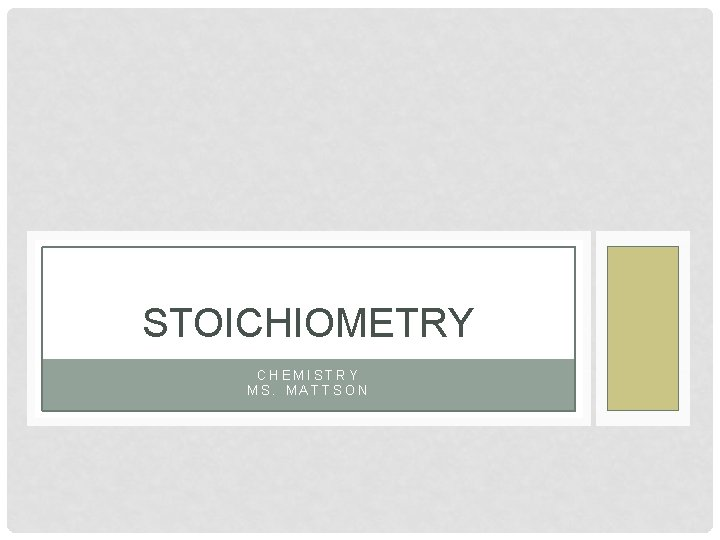STOICHIOMETRY CHEMISTRY MS. MATTSON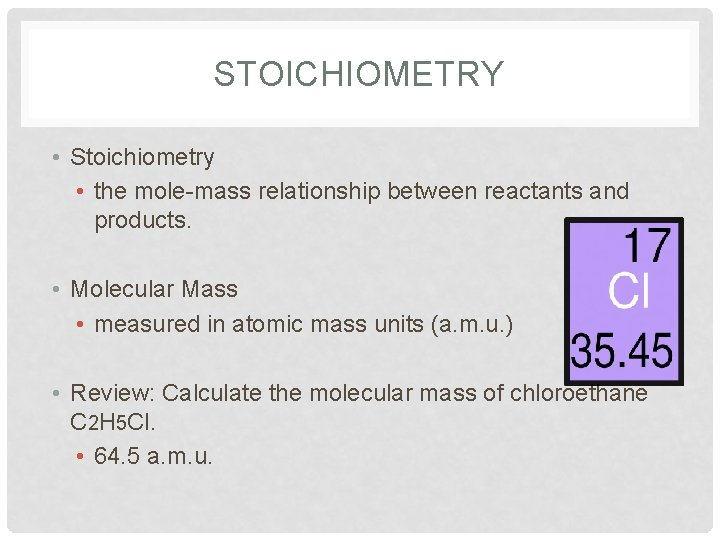STOICHIOMETRY • Stoichiometry • the mole-mass relationship between reactants and products. • Molecular Mass • measured in atomic mass units (a. m. u. ) • Review: Calculate the molecular mass of chloroethane C 2 H 5 Cl. • 64. 5 a. m. u.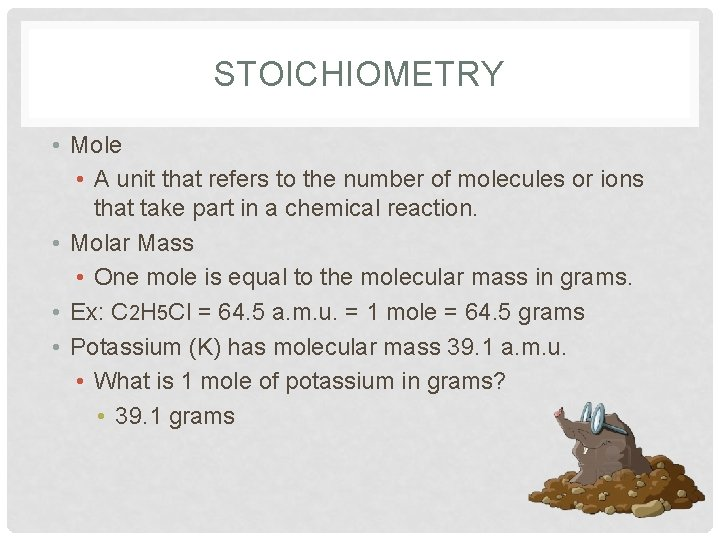STOICHIOMETRY • Mole • A unit that refers to the number of molecules or ions that take part in a chemical reaction. • Molar Mass • One mole is equal to the molecular mass in grams. • Ex: C 2 H 5 Cl = 64. 5 a. m. u. = 1 mole = 64. 5 grams • Potassium (K) has molecular mass 39. 1 a. m. u. • What is 1 mole of potassium in grams? • 39. 1 grams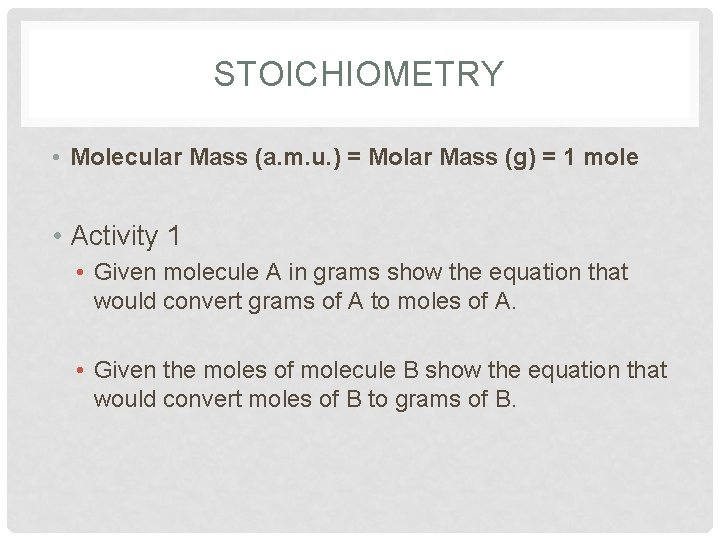STOICHIOMETRY • Molecular Mass (a. m. u. ) = Molar Mass (g) = 1 mole • Activity 1 • Given molecule A in grams show the equation that would convert grams of A to moles of A. • Given the moles of molecule B show the equation that would convert moles of B to grams of B.STOICHIOMETRY • Problem 1 • Convert 15. 0 grams of C 2 H 4 to moles of C 2 H 4. • 0. 536 moles • Convert 0. 536 moles of HCl to grams of HCl. • 19. 6 gramsSTOICHIOMETRY • Molecular Mass (a. m. u. ) = Molar Mass (g) = 1 mole • Activity 2 • Given the reaction 2 A + 3 B A 2 B + B 2 show the equation that would convert the grams of A into the grams of B that are required to fulfill the reaction. • Grams to moles to grams!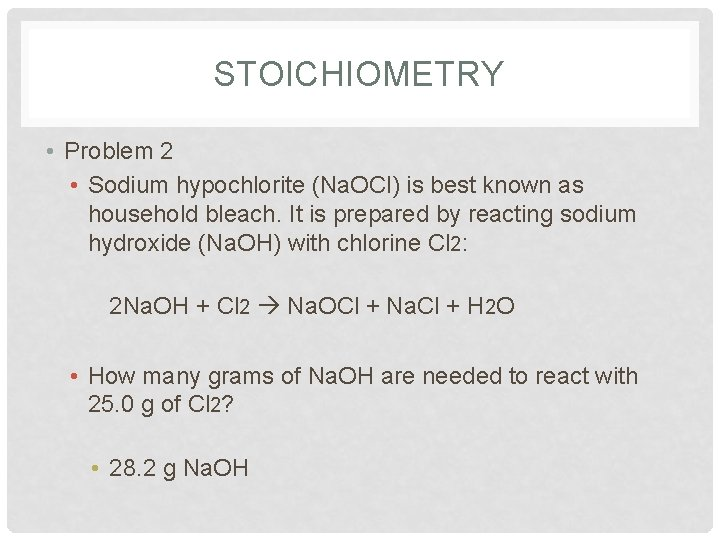STOICHIOMETRY • Problem 2 • Sodium hypochlorite (Na. OCl) is best known as household bleach. It is prepared by reacting sodium hydroxide (Na. OH) with chlorine Cl 2: 2 Na. OH + Cl 2 Na. OCl + Na. Cl + H 2 O • How many grams of Na. OH are needed to react with 25. 0 g of Cl 2? • 28. 2 g Na. OHSTOICHIOMETRY • Problem 3 • Aspirin is prepared by a reaction of salicylic acid (C 7 H 6 O 3) with acetic anhydride (C 4 H 6 O 3) according to the following equation: C 7 H 6 O 3 + C 4 H 6 O 3 C 9 H 8 O 4 + CH 3 COOH Salicylic acid Acetic anhydride Aspirin 138. 1 g 102. 1 g 180. 2 g • How many grams of acetic anhydride are needed to react with 4. 50 g of salicylic acid? • 3. 33 g C 7 H 6 O 3 • How many grams of aspirin will result? • 5. 87 g C 9 H 8 O 4STOICHIOMETRY • Problem 4 • In preparation of iron from hematite Fe 2 O 3 reacts with carbon: Fe 2 O 3 + C Fe + CO 2 How many grams of carbon are needed to react with 525 g of hematite? 59. 2 g C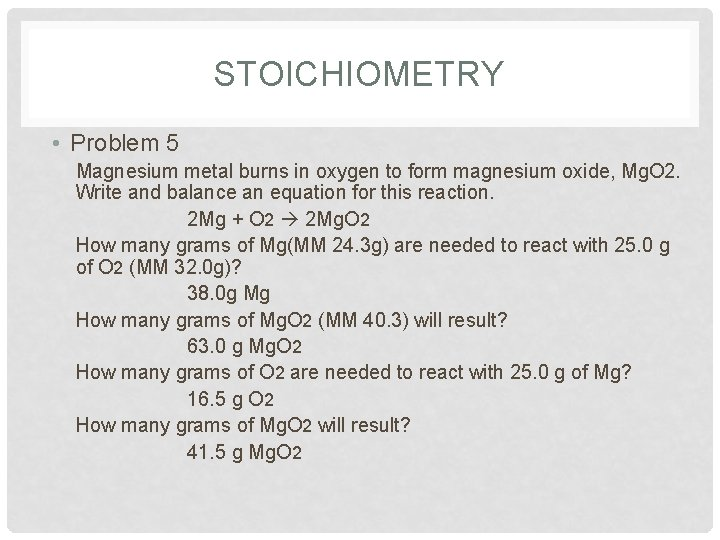STOICHIOMETRY • Problem 5 Magnesium metal burns in oxygen to form magnesium oxide, Mg. O 2. Write and balance an equation for this reaction. 2 Mg + O 2 2 Mg. O 2 How many grams of Mg(MM 24. 3 g) are needed to react with 25. 0 g of O 2 (MM 32. 0 g)? 38. 0 g Mg How many grams of Mg. O 2 (MM 40. 3) will result? 63. 0 g Mg. O 2 How many grams of O 2 are needed to react with 25. 0 g of Mg? 16. 5 g O 2 How many grams of Mg. O 2 will result? 41. 5 g Mg. O 2